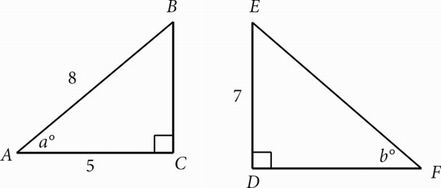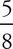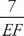# SAT Math Multiple Choice Question 233: Answer and Explanation

### Test Information

Question: 233

8.In the figure above, if sin a = cos b, which of the following is closest to the length of DF ?

• A. 5.6
• B. 8.7
• C. 11.2
• D. 12

B Remember that sin a = cos b means that a and b are complementary angles. Therefore, the two triangles are similar and cos a = sin b as well and you can set up the following equation:=Now that you have solved for EF (11.2), you can use the Pythagorean theorem (a2 + b2 = c2) to solve for DF. 11.22 = 72 + DF2. The correct answer is (B). Alternatively, you could have used the Pythagorean theorem to solve for BC and then set up a proportion between the similar triangles. Just make sure that you recognize that AC corresponds to DE rather than DF.